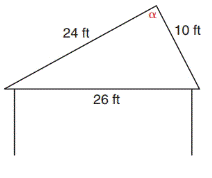Chapter 11.4, Problem 32EElementary Geometry For College St...

7th Edition
Alexander + 2 others
ISBN: 9781337614085

Solutions

Chapter
SectionElementary Geometry For College St...

7th Edition
Alexander + 2 others
ISBN: 9781337614085
Textbook Problem

In Exercises 29 to 34, use the Law of Sines or the Law of Cosines to solve each problem. Angle measures should be found to the nearest degree and areas and distances to the nearest tenth of a unit.Above one room of a house the rafters meet as shown. What is the measure of the angle α at which they meet?To determine

To find:

The measure of the angle α.

Explanation

Formula:

The Law of Cosines

cosα=b2+c2a22bc

cosβ=a2+c2b22ac

cosγ=a2+b2c22ab

Where α, β, and γ is the acute angle of the triangle, and a, b, and c is the sides of the triangle.

Calculation:

Given,

From the figure,

a=26 ft

b=24 ft

c=10 ft

Find α:

The alternative from of the Law of Cosines,

cosα=b2+c2a22bc

Still sussing out bartleby?

Check out a sample textbook solution.

See a sample solution

The Solution to Your Study Problems

Bartleby provides explanations to thousands of textbook problems written by our experts, many with advanced degrees!

Get Started

Finding Derivatives In Exercises 51-62, find f(x). f(x)=x(x2+1)

Calculus: An Applied Approach (MindTap Course List)

In Exercises 39-54, simplify the expression. (Assume that x, y, r, s, and t are positive.) 40. (49x2)1/2

Applied Calculus for the Managerial, Life, and Social Sciences: A Brief Approach

In Exercises 110, find the graphical solution to each inequality. y3

Finite Mathematics for the Managerial, Life, and Social Sciences

True or False: i + j is a unit vector in the direction of 5i + 5j.

Study Guide for Stewart's Multivariable Calculus, 8th

The arc length function for y = x2, 1 x 3 is s(x) = a) 1x1+4x2dx b) 1x1+4t2dt c) 1x1+t4dt d) 1x1+4t4dt

Study Guide for Stewart's Single Variable Calculus: Early Transcendentals, 8th